Sunday 20th September 2020

CBSE Guess > Papers > Question Papers > Class XII > 2003 > Mathematics > Compartment Delhi Set -II

MATHEMATICS—2003 (Set II—Compartment Delhi)

Note: Except for the following questions, all the remaining question have been asked in Set I .

SECTION - A

Q. 6. Evaluate: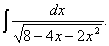Q. 8. Solve for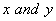, given that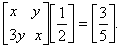Q. 10. The two lines of regression are given by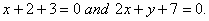Find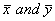and the co-efficient of correlation between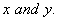Q. 12. Events A and B are given to be independent. Find P (B), if it is given that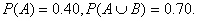Q. 15. Evaluate:Q. 18. Using integration, find the area of the triangle ABC, whose vertices have coordinates: A (3, 0), B (4, 6) and C (6, 2).

SECTION - B

Q. 22. Find the value of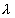so that the two vectorsare
(i) Parallel,
(ii) Perpendicular to each other.

SECTION - C

Q. 21. For a near normal distribution, Kari Pearson's co-efficient of skewness is 0.6. The standard deviation of the distribution is 12 and the mean is 45. Find its mode and median.

 Mathematics 2003 Question Papers Class XII Delhi Outside Delhi Compartment Delhi Compartment Outside DelhiSet ISet ISet ISet ISet IISet IISet IISet IISet IIISet III

CBSE 2003 Question Papers Class XII